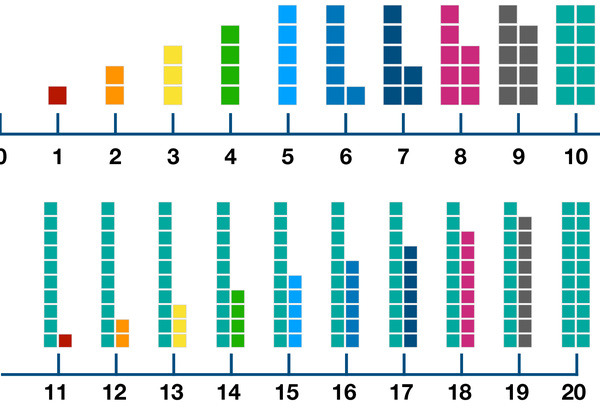# Number Blocks: Number Line to 20In math, a number line is a straight line with numbers shown as specially-marked points evenly spaced on the line. A number line is usually represented horizontally, and it can go forever in each direction. The numbers go up from left side to right side on the number line. Number line should be introduced to kids at a very young age. It can help kids build their number sense. It also provides a mental strategy for addition and subtraction. Number line can be used in math study throughout all the time of primary school.

This worksheet provides a number line from 0 to 20, and each number is also illustrated by the corresponding number of blocks. Parents can use it to introduce number lines to kids. It is a great tool for kids to visualize numbers, compare and connect numbers, practice addition by moving to the right, and subtraction by moving to the left.